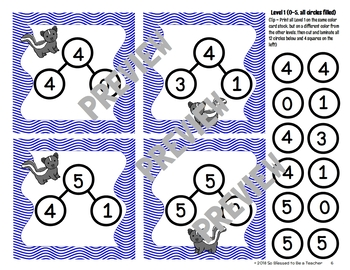# Kindergarten / First Grade Math Center Game - Stinky Number Bonds!Subject
Resource Type
File Type
PDF (1 MB|19 pages)
Standards
\$2.25
• Product Description
• Standards

This is a silly, fun game that will give your kindergarten and first grade students practice with number bonds, addition, and subtraction. This game allows for differentiation and would be perfect for math centers!

Students work in groups of 2-4 to play this game, which will allow them to match numbers to number bonds and use the number bonds to say a number sentence. (But here's the fun part - they get to say the number sentence while holding their nose - like they smell something stinky!)

This game includes 6 "levels," allowing you to use the same game for different students. Levels 1 and 2 deal with numbers up to 5, Levels 3 and 4 involve numbers up to 10, and Levels 5 and 6 contain numbers up to 19 (split into 10 + ones).

Your students will love this opportunity to turn number bonds into number sentences and number sentences into a fun time! ☺

Thanks a million for visiting my store! Please follow me to stay informed about freebies, new products, and sales! May God bless you and your students! ☺

If you purchase this product and find it useful, please consider leaving feedback! Remember, whenever you leave feedback on a paid product, you can earn credits that can be used to reduce the cost of a future Teachers Pay Teachers purchase!

Determine the unknown whole number in an addition or subtraction equation relating three whole numbers. For example, determine the unknown number that makes the equation true in each of the equations 8 + ? = 11, 5 = ▯ - 3, 6 + 6 = ▯.
Add and subtract within 20, demonstrating fluency for addition and subtraction within 10. Use strategies such as counting on; making ten (e.g., 8 + 6 = 8 + 2 + 4 = 10 + 4 = 14); decomposing a number leading to a ten (e.g., 13 - 4 = 13 - 3 - 1 = 10 - 1 = 9); using the relationship between addition and subtraction (e.g., knowing that 8 + 4 = 12, one knows 12 - 8 = 4); and creating equivalent but easier or known sums (e.g., adding 6 + 7 by creating the known equivalent 6 + 6 + 1 = 12 + 1 = 13).
Understand subtraction as an unknown-addend problem. For example, subtract 10 – 8 by finding the number that makes 10 when added to 8.
Fluently add and subtract within 5.
For any number from 1 to 9, find the number that makes 10 when added to the given number, e.g., by using objects or drawings, and record the answer with a drawing or equation.
Total Pages
19 pages